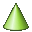# Cone

Create a cone.

On the Construct tab, in the Create Solid group, click theCone icon.
Tip: Press C,5 to use the shortcut key.Base centre (B) The centre of the base of the cone. Base radius (Rb) The radius of the cone base (parallel to the UV plane). Height (H) The cone height in the N direction measured from B. Top radius (Rt) The radius of the cone top (parallel to the UV plane).Base centre (B) The centre of the base of the cone. Top centre (T) The centre of the top of the cone. Base radius (Rb) The radius of the cone base (perpendicular to the line from B to T). Top radius (Rt) The radius of the cone top (parallel to the UV plane).Base centre (B) The centre of the base of the cone. Base radius (Rb) The radius of the cone base (parallel to the UV plane). Height (H) The cone height in the N direction. Flare angle (A) The growth angle measured from the N axis.Base centre (B) The centre of the base of the cone. Top centre (T) The centre of the top of the cone. Base radius (Rb) The radius of the cone base (perpendicular to the line from B to T). Flare angle (A) The growth angle measured from the line defined between B to T.